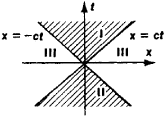# light cone

Also found in: Wikipedia.

## light cone

The representation of a light flash in spacetime. In three-dimensional space the wavefront of a light flash would be a sphere centered on the emitting point and growing in radius at the speed of light. In spacetime (with one spatial coordinate suppressed) the vertex of a cone represents the time and place at which a flash is emitted and the cone itself describes the history of the propagating flash. More generally a light cone defines the regions of the Universe accessible from a given point in space and moment in time, i.e. from a given position in spacetime. This position is called an event and is the vertex of the light cone.

## Light Cone

a concept used in describing the geometric properties of four-dimensional space-time in the special and general theories of relativity. The light cone corresponding to a given point in space-time is the three-dimensional surface in this four-dimensional space formed by the set of world lines of freely propagating light signals (or of any particles with zero rest mass) passing through the point, which is the vertex of the cone. Thus, to every point of four-dimensional space-time there corresponds a light cone.

In the case where the special theory of relativity is valid, the geometry of space-time is a pseudo-Euclidean geometry, called Minkowski geometry, in which all points of space-time are equivalent. Therefore, it is sufficient to consider a light cone with its vertex at the origin of coordinates O, where x= 0, y = 0, z = 0, and t = 0; where, x, y, and z are the space coordinates and t is the time coordinate. The equation of a light cone with vertex at O has the form x2 + y2 + z2c2t2 = 0, where c is the speed of light in a vacuum. This equation is invariant with respect to Lorentz transformations. Points (events) with x2 + y2 + z2c2t2 and with t > 0 or t < 0 form what can be called the future and past interiors of the light cone—regions I and II, respectively, in Figure 1. Events with x2 + y2 + z2 > c2t2 form region III outside the light cone.Figure 1

The intersection of a light cone with the plane y = 0, z = 0 is illustrated in Figure 1. The light cone intersects this plane along the straight line x = ± ct. Events A lying in region I form the absolute future with respect to the event O. Event O can exert a direct influence on any event A, since signals or interactions can connect O with A. Correspondingly, events B in region II form the absolute past for event O. Any event B can affect event 0, because signals from B can reach O. Events in region III cannot be connected with O by any interaction, since no particles or signals propagate faster than light.

Thus, the light cone separates events that can be in a causal relationship with O from events for which a causal relationship is impossible—this separation is the fundamental significance of the concept of the light cone. An observer located at O can know only of events in region II and can influence only events in region I.

In the presence of gravitational fields the world lines forming the light cone are not straight lines. In this case, the properties of the light cone near the vertex are the same as in the special theory of relativity. In the large, however, the properties are different, since the geometry of space-time is not pseudo-Euclidean.

I. IU. KOBZAREV

## light cone

[′līt ‚kōn]
(relativity)
The set of all points in space-time that are reached by signals traveling at the speed of light from a specified point, or from which signals traveling at the speed of light reach that point. Also known as null cone.
References in periodicals archive ?
This is the second generation of Lucibel LiFi luminaire products, which enables internet access through light emitted from the light cone, based on PureLiFi technology.
In this work, we estimate the strong coupling constants of [[OMEGA].sup.0.sub.c] [right arrow] [[XI].sup.+.sub.c][K.sup.-] in the framework of light cone QCD sum rules.
The light's LEDs are configured at a 20-degree angle to create an ultra-wide light cone that illuminates a broad area, eliminating the need to rotate it for greater coverage.
We also studied the problem from some aspects (Sapar, 1977), but the problem how to connect the past horizon on a light cone causally with the Planck units remained unsolved.
Philosophers of physics use a light cone as a visual aid for understanding this cosmic speed limit and the trajectory of events that are knowable, as they propagate outward in waves through the geometry of spacetime.
Then [[gamma]'.sub.1] is contained in the intersection of the light cone [Q.sup.4.sub.2,0] with the hyperplane [{[e.sub.0]}.sup.[omega]].
The installation step into my light cone emerged during the Art and Light Project as a series of images captured from interactive projections producing caustic effects.
The Baader f/2 Highspeed Filter series combats this problem by shifting the passband of each H[alpha], O III, and \$ II filter lower to accommodate the steep light cone of telescopes with extremely fast f/ratios between f/1.8 and f/3.5.
It works by dividing the LED light cone into 12 segments that switch off individually and automatically as required when a forward facing, on board camera detects other vehicles, cyclists or pedestrians ahead or approaching from the opposite direction.
His argument for an ontological and inhomogeneous past-present-future structure ("ppf") within a light cone logically entails that, mutatis mutandis, more than that single light cone has the same ppf structure.
The set of light-like vectors Q = {x [member of] V | B(x, x) = 0} form a cone called the light cone.

Site: Follow: Share:
Open / Close